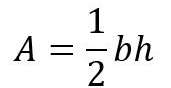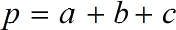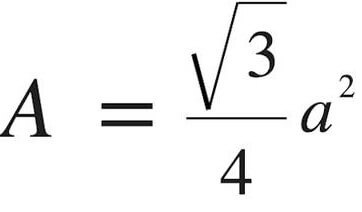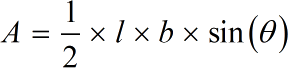Request a Tool

Triangle Area Calculator

Triangle calculator use to calculate area and perameter of a triangle quickly.

Area
0

Formula• a = Area of the triangle
• b = Breadth of the triangle
• l = Length of the triangle

Defination / Uses

A triangle is a flat figure with three angles and straight sides. The sum of all the inside angles is 180 degrees. A triangle is a polygon with three vertices (corners) and three sides (edges) (line segments). ABC refers to a triangle with vertices A, B, and C.

Perimeter
0

Formula• a = Value for side a of triangle
• b = Value for side b of triangle
• c = Value for side c of triangle

Defination / Uses

The whole length of the triangle's boundary is specified as its perimeter. A triangle is a three-sided polygon with distinct varieties dependent on the lengths of its sides and angles.

Output

Area of a equilateral triangle
0

Formula• a = Area of equilateral triangle
• h = Height of triangle

Defination / Uses

A triangle with all three sides equal is called an equilateral triangle. That is, all three internal angles are equal, and the only value conceivable for each is 60 degrees. An equilateral triangle's perimeter is 3a.

Triangle SAS
0

Formula• a = Area of triangle SAS
• l = Length of triangle
• b = Breadth of triangle
• θ = Angle of a side for triangle

Defination / Uses

A triangle is a flat figure with three angles and straight sides. The sum of all the inside angles is 180 degrees. A triangle is a polygon with three vertices (corners) and three sides (edges) (line segments). ABC refers to a triangle with vertices A, B, and C.

How to calculte area of a triangle?

There are only two basic steps.

• First select the option from dropdown. What you need to calculate.
• Then add the other values in their corresponding fields.